﻿ Evaluation of the Precision and Accuracy of Multiple Air Dispersion ModelsPublications are Open
Access in this journal
Article Versions
Export Article
• Normal Style
• MLA Style
• APA Style
• Chicago Style
Research Article
Open Access Peer-reviewed

### Evaluation of the Precision and Accuracy of Multiple Air Dispersion Models

Frank Gronwald , Shoou-Yuh Chang
Journal of Atmospheric Pollution. 2018, 6(1), 1-11. DOI: 10.12691/jap-6-1-1
Published online: February 10, 2018

### Abstract

Determining the level of air pollution is a modern day necessity for government regulators and industrialized sources. Air dispersion models are often used to determine the concentration of a pollutant. However changing conditions and several assumptions made by the models limit their accuracy at various times. The objectives of this research are the evaluation of four different air dispersion models (Gaussian Plume, Variable K Theory, Box, and AFTOX) as well as developing a more efficient method of evaluating air dispersion models. In the process of determining their performance, the change in accuracy was measured through RMSE calculations and the change in precision was measured through calculating the Brier Score. It was found that in the prediction of NO2 Variable K Theory and Gaussian Plume both models produced average reduction of 21.2% of mean RMSE from the other models. The Variable K model also proved to be most precise with a 8.9% lower Brier Score than the next best model.

### 1. Introduction

There is a great need to determine the concentration and pathways of airborne pollutants due to the health and environmental hazards they cause to arise. There are several air dispersion models in use today and each of them has their own strengths and weaknesses. The weaknesses are the areas where the model is not able to produce accurate results. Thus several models can be given the same data and produce very different results. This leads to a great deal of confusion as to which model to choose under certain conditions. One of the principal problems with the current air dispersion models is the amount of error contained within their forecasts. The errors are produced and may consist of a multitude of sources, such as measurement error and numerous estimated and calculated parameters used in each model’s algorithm that may result in a different value than the actual one. This leads to issues where the end user is unsure which model to apply under specific conditions. The cumulative effect of the model’s internal error will typically affect the predicted concentration as well as it will have an impact on many other features including the calculated mean, standard deviation, and length of predicted exposure. The objectives of this study are to compare and contrast a set of air dispersion models as well as to determine a better alternative method of evaluating air dispersion models.

The concept of creating models for the estimation of the concentration of specific air pollutants was started shortly after World War I when army researchers from England attempted to predict the path of poison gas chemicals when emitted over the ground troops and how it would be affected by temperature and changing weather. Sutton 1 presented results of much of that work in his book. When scientists gained further knowledge of the atmosphere in the 1960’s the pace of pollutant detection gained momentum and more promising air dispersion models began to appear which could be used for regulatory purposes. The works produced by Pasquill 2 and Stern 3 discuss the various experiments performed over that time. Today the staggering level of pollutant tracking and the increasing size and scope of the models being developed almost forces researchers to comb specialized journals and conference proceedings on a daily basis to look for new developments 4, 5, 6.

One of in drawbacks of using any air dispersion model is the amount of error in the estimation of the pollutant concentration that gets produced. This error mostly arises from the inherent difficulty of using a relatively simple modelling framework to determine the concentration profile which is influenced by very complex and variable stimuli 7. In the process of calculating the concentration of a pollutant there exist many factors that contribute to overall derived error. Some of the more prominent sources of error for a particular model include: the development of the mathematical formula used by the model, the quality of the initial parameters used in the calculation, the number of pollutant sources, and the complexity of the atmosphere 8.

Another prominent source of error is commonly found during the collection and analysis of air samples. The concentration data obtained from the air samples gathered at the same testing site but at different time periods could vary widely 9, 10. The sampled data could differ by 10 or even 100 times as much due to changes in the wind direction, atmospheric stability, emission level, reactivity of the pollutant, or the current pollutant phase 11.

However, there are also many sources of error that end up being too costly and impractical to obtain a full or sometimes a partial estimation 12. Some of these instances include: missing some or all of the data necessary for calculating the magnitude of imprecision from the measurements, the available data being insufficient, or lacking the knowledge about how exactly this lack of information is related to the uncertainty calculation 13. One example of this type of error is created through the relationship between the SO2 and NO2 concentrations and the sulphur and nitrogen content of the fuel source for the smokestack. Since the fuel source is typically not fully heterogeneously mixed, the SO2 and NO2 concentrations will vary 7. The SO2 and NO2 concentrations may also be influenced through the various manufacturing processes being run 14. Human error and unpredicted events are also sources of unknown error.

The main corrective solution that is used to minimize the error is to create adjustments in the model calculations 13. By calibrating the model against known results it is hoped that error may be minimized although some bias is introduced within the process. One of the possible workarounds is to use several models with the objective that some of the error will cancel itself out.

### 2. Methodology

This study examines the following air dispersion models in use today (Gaussian Plume, Variable K Theory, AFTOX, and Box) and attempts to identify their strengths and weaknesses. This set of models was chosen for a multitude of reasons. These four models are most closely related and share common input parameters. Many of the meteorological parameters used in the more complex models were not included with the data sets used, thereby eliminating them from consideration. Another factor is in the assumption of steady state conditions.

2.1. The Gaussian Plume Model

The Gaussian Plume Model is a mathematical model used to approximate the concentration of one specific air pollutant originating from a single point source. The model assumes steady state conditions. Dispersion is assumed to occur in three directions: downwind (x direction), crosswind (y direction), and vertically (z direction). Sutton 1 developed the following equation: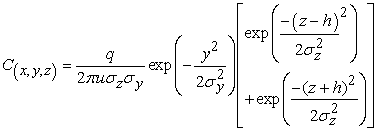(1)

whereis the concentration (in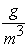) at a point which is located x meters downwind of the source, y meters away from the centerline of the plume and z meters above the ground; q is the flow rate of the pollutant, (in); u is the instantaneous wind velocity (in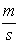); h is the height of the source above ground level (in m); andandare the standard deviations of the concentration of the yz plane 15. The horizontal dispersion parameter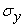is calculated using the power law 2.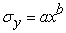(2)

where a and b are obtained from the values discerned from the Pasquill Stability Table as shown in Table 1.

The Pasquill Stability Table was developed as a way to classify the atmospheric conditions by using a single letter. These letters range from Extremely Unstable (A) to Moderately Stable (F) and they are based on many conditions including: time of day, wind speed, temperature, and turbulence 2.

The vertical horizontal dispersion parameter,, is found in a very similar way with the exception of being more dependent on the downwind distance away from the source (in the x direction). It is also calculated using a form of the power law 3.(3)

where c and d are coefficients based off of the Pasquill Stability Table as displayed in Table 2.

The Variable K Theory Model was essentially the steady state dispersion of a pollutant and was derived from the equation for the Advection Dispersion model 16: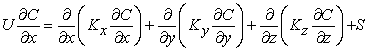(4)

where C is the mean concentration (g/), S is the source term (g/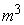),, andare the eddy diffusion coefficients in the x, y, and z directions respectively, and U is the mean wind velocity in the x axis (m/s). The solution of Eq (4) depends on the values of U, K and S. Sharan and Yadav 17 found that for optimum performance the value for U could either be a constant or determined through a power function of z, while the K values could either be constant or a function of their position, and S is based on the position of the source relative to the coordinate system. For the purpose of this study, the Variable K Theory model was created and utilized under the conditions of the Advection Dispersion equation with U being kept constant at a non-zero value. The K values originated as linear functions of the downwind distance based on Taylor’s theory for small travel times such that 17:(5)

where α, β, andrepresent the turbulence parameters and were found to vary with stability 16. By implementing these conditions, the fundamental equation is formulated to 17: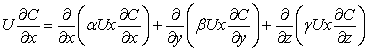(6)

withwhere U is the mean wind velocity and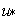is the friction velocity (m/s) 17.

The Variable K Theory model was composed from two special cases of the Advection Dispersion Model. The first special case required isotropic diffusion and calm conditions (U=0) 18. Since the other air dispersion models used in this work require a wind velocity, the no wind special case was omitted from the analysis of this study. The second special case required isotropic diffusion and a wind velocity of under 218. The following equation was used to calculate the concentration at a given point 16: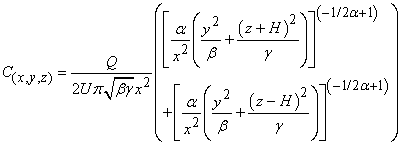(7)

with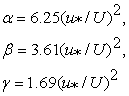where Q is the pollutant emission rate (g/s), and H was the effective stack height (m).

2.3. The AFTOX Model

Kunkel 19 created AFTOX stemming off of a project where he had upgraded and enhanced an older model called SPILLS. SPILLS was developed by the Shell Oil Company 20. The AFTOX model was based on the Gaussian Puff equation, which was used to determine the dispersion of a puff of air over time. The Gaussian puff equation used the scientific law of conservation of matter during its calculations so it did not apply the principle of decay or settlement. This often led to the AFTOX model making higher predictions than other models. To model steady state pollutant flows, AFTOX assumed there were four puffs released every minute 19. Another assumption made was that the distribution of concentration within the puff was Gaussian. The Gaussian puff equation is as follows 21: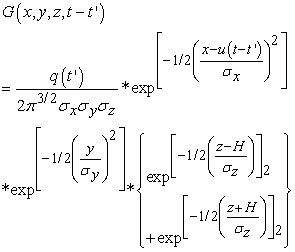(8)

where G is the concentration of the puff at a given point (x,y,z) and time (t - t'), q is the rate at which the puff is released (g/s). The parameters:,,are the standard deviation of the concentration in the x, y, and z directions, respectively. The variable t is the total elapsed time since the pollutant release, and t' is the time of emission of the puff. Thus t - t' is the time the puff had travelled since it was emitted 22. The variable u is the wind speed at 10 m, and H is the height of the source (m). It is assumed that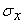=to produce a circular horizontal puff cross-section 19. The values ofandare determined through a set of complex calculations which were discussed in detail by both Zettlemoyer 20 and Kunkel 21.

2.4. The Box Model

The box model operated on the principle that the emitted plume from a point source would expand so that it would fill the entire surface area of a theoretical cube having a width y and a height z 23. This required the calculation of the average concentration of the plume for every location downwind. If an amount of pollutant exiting the box is equal to that entering the box then the box volume is directly proportional to the wind speed. The equation for the calculation of the box concentration is 24: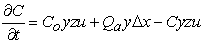(9)

where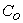is the initial concentration entering the box (g/s), C was the pollutant concentration exiting the box (g/s), u is the instantaneous wind velocity (m/s),is the downwind distance away from the source (m),is the plume flow rate inside the box (m^3/s), and y and z are the box width (m) and height (m) respectively. When the case of steady state concentration is considered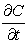becomes zero and Eq. (9) adjusts to 24:(10)

Williamson 24 discovered there was essentially an inverse relationship between the concentration and both wind velocity as well as mixing height. That is due to the ability of both to expand the theoretical box as they increased.

Since the air dispersion models are run under steady state conditions, there are common procedures to test their performance, i.e., the Root Mean Square Error and the Brier skill score.

2.5. Performance Evaluation

After the calculations were performed for all the selected models, the final step was to evaluate their performance. The evaluation for each case study utilized two popular methods in determining the accuracy of superensemble and its member models: the Root Mean Square Error (RMSE) and the Brier Score. The formula to calculate RMSE is given below:(11)

where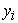is the calculated concentration,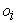is the measured concentration, and n is the number of points.

The Brier Score is an effective method of analysing a model’s performance because it is really a probability forecast. This means that it is subject to being verified through analysing the joint statistical distribution of forecasts and observations 25. A probabilistic forecast is one that estimates the probability of occurrence of a chosen event ε. One of the most widely used methods for the verification of probability forecasts is the Brier skill score. A Brier skill score is calculated by first selecting an event ε that either happened at realization k or did not 26. The value of the function was o(k) = 1 if ε occurred, and o(k) = 0 if it did not. This forecast of occurrence with probability was represented as f(k). The Brier score is defined as 27: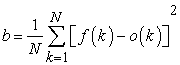(12)

where the index k refers to the forecast–observation pairs and n is the total number of such pairs within the dataset 28. The lowest possible value of the Brier score is zero, and it can only be obtained by a perfect forecast. The Brier score can be further broken down into three terms: reliability, resolution, and uncertainty, as shown below 29: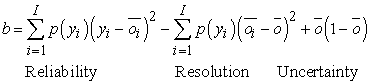(13)

where= (1/nnk=1 o(k) and is the unconditional mean frequency of occurrence of the event ε, and i is the number of discrete categories. The frequency with which forecasts of yi were issued is p(yi) 26.

The reliability term evaluates the statistical accuracy of the forecast. A perfectly reliable forecast is one for which the observed conditional frequency oi is equal to the forecast probability (or the overall forecasts for y-percent chance of ε, ε occurred in y percent of the times). The resolution term refers to the distance between the forecast frequency and the unconditional climatological frequency. Forecasts that were always close to the climatological frequency exhibited good reliability, because the forecast frequency matched the observed frequency 30. However these forecasts suffer from poor resolution, because they are not able to distinguish between different regimes. The uncertainty term is a measure of the variability of the system and is not influenced by the forecast 29.

The purpose of performing the Brier Score calculation was to obtain an additional weight for each model to compensate for the variation of model’s accuracy 30. For each case study, the event was hereby defined as a concentration found to be above the threshold level, which was set at a fixed level depending on the initial parameters and the levels mandated by the EPA National Ambient Air Quality Standards (NAAQS) for each air pollutant.

Each calculated concentration from the model output was compared to the threshold value to determine whether an event (concentration value exceeding the threshold value) had occurred. The observed values were also compared to the threshold to determine if an event occurred 31. After this process had been completed, the event or non-event result for each model was compared with that of the observed. In this study a threshold value of 14.7 ppm was used which was derived from the worst case scenario of the maximum one time emission that could be allowed. The EPA guidelines allow for an annual maximum average of 0.53 ppm not to be exceeded 32. If there were multiple air pollutant emissions the threshold value would be lower.

Because the accuracy of each model had a tendency to vary, it was then prudent to assign an additional weight based on the probability forecast density. The probability of an eventwas defined as 29:(14)

where=0 if the event did not occur and=1 if the event occurred. Pagowski et al. 33 found that these new weights also needed to be normalized so the total was 1. The weights were calculated during the calibration period using the following equation 34:(15)

wherewas the sum of the hit rate for predicting the event occurring and the hit rate for predicting the event not occurring. The value for k was a fixed constant that Cane and Milelli 35 found to provide the optimum level of precision at a value of 3.

The value for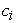can be found using the following equation 36 :(16)

where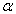represents the number of events that are both predicted and observed,represents the amount of events that are predicted but not observed,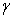is the number of events observed but not predicted, andrepresents the number of events that were neither predicted nor observed.

One of the advantages of this method to define the weights is that it is event dependent and thus can be better represent the relative trustworthiness of the different models with respect to the chosen event 37. With either method the weights are subject to variability in space so the different models may have varying relative contributions to the total probability depending on the location of each data point 38.

2.6. Data Collection

The data sets utilized in these experiments originated from the EPA website. They were located on the Preferred/Recommended Models web page in the AERMOD modelling section under the Model Evaluation Databases 39. During this study there were data sets available for 17 locations. Each location was contained within a downloadable file which encompassed several more files that included information pertaining to the input data, initial AERMOD and AERMET settings, buildings or other obstructions, and observed pollutant concentration measurements 40. These initial conditions were used for each member model calculation. The observed concentration measurements became utilized in both the superensemble formation as well as the RMSE calculations.

Generally, there were two main air pollutants which had concentration data available: NO2 and SO2. Also the data sets only considered two types of terrain: flat and mountainous no matter whether downwash conditions were present 41. For this research data sets only using the same pollutant, downwash condition, and type of terrain were utilized in the same experiment.

In most of the data sets there were three measurement runs taken at different times within a short interval. To simulate steady state conditions these values were combined to obtain a mean concentration value 42. One caveat to this process was that on several occasions the concentration values from one measurement run were found to have a significant deviation from the other measurement runs for that location 43. When that occurred, the concentration values from that run were considered an outlier and rejected so that the mean concentration calculated would be originated from the two data sets that were close together 44. The initial parameters from the three data sets were also combined to obtain a mean value. These mean values for both the initial parameters and measured concentrations for the data sets were used in the case study. This study determined the effectiveness of the models in the prediction of the NO2 emissions.

### 3. Results and Discussion

This case study looked at the performance of the member models using two of the data sets available from the EPA Model Evaluation database. The Westvaco data set was used for calibrating the models and the Baldwin data set was used for the evaluation phase.

The Westvaco dataset originates from the Westvaco Corporation’s pulp and paper mill in Luke, Maryland. The original goal was to acquire data in a complex terrain setting. It was located in the Potomac River valley in western Maryland 45. Factors that impacted the data include the location of the facility, topographic features, and locations of the ambient monitors and on-site meteorological data towers. A single 185 m stack was modeled for this evaluation. There were 11 NO2 monitors surrounding the facility, with eight monitors well above stack top on the high terrain east and south of the mill at a distance of 800 - 1500 meters 46. Two monitors, were placed at elevations approximately equal to stack top and were 900 meters NNW and 3300 meters NE, respectively. One monitor at Bloomington was located 1500 meters northwest at about stack base elevation. Hourly meteorological data was collected between December 1980 and November 1991 at three instrumented towers: the 100-meter Beryl tower in the river valley about 400 meters southwest of the facility; the 30-meter Luke Hill tower on a ridge 900 meters north-northwest of the facility; and the 100-meter Met tower 900 meters east-southeast of the facility on a ridge across the river 45.

The Baldwin data set originates from the evaluation of the Baldwin Power Plant

Which resides in a flat terrain setting of southwestern Illinois. The terrain was found to slope gradually upward on the east side of the facility. Three 250 meter stacks have an alignment that is approximately north-south with a horizontal spacing of about 100 meters between them 46. Building widths and heights were included in their evaluation. Although the stacks were found to be slightly less than the Good Engineering Practice height, there was sufficient momentum rise present to avoid building downwash effects under most meteorological conditions 46. There were 10 NO2 monitors surrounding the facility ranging in distance from two to ten kilometers. On-site meteorological data from the Baldwin field study covered the period from April 1, 1982 through

March 31, 1983 and consisted of hourly wind speed, wind direction, and temperature measurements taken at10 meters and hourly wind speed and wind direction at 100 meters 45. Both the National Weather Service upper air soundings and hourly surface observations were included by EPA.

The initial parameters required for the operation of each model were obtained from the initial conditions given in each data set. These initial values are given in Table 3. The NO2 concentration data that had been collected from both the Westvaco and Baldwin sites was organized into three separate files, which contained the concentration measurements WVA01, WVA03, and WVA24 and BAL01, BAL03, and BAL24 originating from slightly different time periods. The concentration profiles of the three Westvaco measurement runs are given in Figure 1 and the three Baldwin measurement runs in Figure 2. The data from all three Westvaco measurement runs as well as their initial conditions were then averaged into a single data set to simulate an air pollution concentration profile under steady state conditions. However in the Baldwin measurement runs, the data from the BAL01 run was significantly different from the other two and was treated as an outlier. This means that only two measurement runs and their initial conditions were averaged to simulate a single collection under steady state conditions.

The initial conditions of each separate time period are given in Table 3. Some of the initial parameters such as stack height and diameter had values that stayed constant throughout. Other parameters such as wind velocity and exit temperature had slight variations. One additional point of reference is the changing of the concentration from the given model unit (g/m3) to (ppm). The conversion factor is 1:1 and the latter provides a more asthenic appearance in the upcoming figures and tables.

The models were calibrated using the Westvaco dataset to produce calculations that were as close to the measured values as possible and are shown in Figure 3. The calibrated models were then used to predict the NO2 concentration at the Baldwin location.

• Figure 1. NO2 concentration the profile for Westvaco location
• Figure 2. NO2 concentration profile for the Baldwin location
• Figure 3. Calibrated NO2 concentration profile for the Westvaco location
• Figure 4a. Measured NO2 concentration profile for the Baldwin location
• Figure 4b. NO2 concentration profile for the Baldwin location calculated by the Gaussian Plume Model

The measured concentration of NO2 is shown in Figure 4a. There are measurements taken in the crosswind (y) direction for a maximum distance of 5m away from the centerline. This would factor in for the calculated concentration values for each model which are shown in Figure 4b, Figure 4c, Figure 4d, and Figure 4e. From Figure 4d it can be seen that the AFTOX model has both higher sloping rates and a higher peak that the other models. The Gaussian Plume model also started producing a reduction of accuracy at the crosswind distance increased due to the Pasquill Stability Parameters only being applicable in the downwind direction.

• Figure 4c. NO2 concentration profile for the Baldwin location calculated by the Variable K Model
• Figure 4d. NO2 concentration profile for the Baldwin location calculated by the AFTOX Model
• Figure 4e. NO2 concentration profile for the Baldwin location calculated by the Box Model

Since Turner and Shulze 8 found the concentration is typically the highest at the centerline, this study concentrated its focus in that region. Figure 5a and Figure 5b display the centerline profile of the calculated concentrations from the models as well as the NO2 concentrations that had been previously measured. The models are shown in two sets of graphs to give a clear and easy to understand picture of what is transpiring along the concentration run. Figure 5 displays the output of all the models in the same graph. It is apparent from these graphs that the Variable K Theory and the Gaussian Plume models are prone to under-prediction while the Box model had a tendency to over-predict the measured values.

• Figure 5a. Calculated NO2 concentration profile at the Baldwin location
• Figure 5b. Calculated NO2 concentration profile at the Baldwin location
• Figure 5c. Calculated NO2 concentration profile at the Baldwin location

Once the calculated concentrations were obtained from each model, they could be compared to the known measured values. Figures 6a and 6b show the calculated centerline RMSE for each model. The highest RMSE for each model tends to be near the peak. This is to be expected as the peak is the portion of the concentration run with the greatest potential for variance. The average RMSE for the downwind run was calculated and is shown in Table 4. While there were specific data points where each model had the lowest RMSE, the overall goal was to find the model with the highest consistency. Both the Gaussian Plume and Variable K Theory model produced lowest errors at just over an average of 24 ppm. There were several factors that play a part in the reduced accuracy of the other models. The AFTOX model suffers from poor performance in the first moments of pollutant release up until the peak concentration was reached. The Box model has a tendency to engage in the highest amounts of over-prediction in the moments after the peak concentration was reached which increased the mean RMSE.

• Figure 6a. Calculated RMSE profile for the NO2 concentration at the Baldwin location
• Figure 6b. Calculated RMSE profile for the NO2 concentration at the Baldwin location

In addition to the RMSE, the Brier Score is also calculated for each model and is displayed in Table 5.

The first four lines of Table 5 provide a snapshot of the performance of the member models. The hit rate, normalized weight, and probability all factored into the Brier Score calculation. α represents the number of calculated and measured observations that are both above the threshold value. β shows the number of observations where the measured value is below the threshold value while the calculated value obtained is above the threshold value. This is generally known as a false positive. γ shows the number of observations where the measured value is above the threshold value while the calculated value obtained is below the threshold value. This is generally known as a false negative. δ shows the number of calculated and measured observations that are both below the threshold value. By comparing the total sums for each model of α and β with those found in γ and δ, it was found in this instance that the Variable K and the Gaussian Plume have a tendency to slightly under predict while the AFTOX and Box models have a significant bias towards over predicting. This is expressed in the calculated probability value for each model. The Box model ended up generating a large amount of false positive readings which led to the high Brier score. The most precise model was found to be the Gaussian Plume one, which benefitted from both a high hit rate and lowest probability to generate a 8.9% reduction from the next most precise model (Variable K).

### 4. Conclusion

There are two main findings of this research. The first one being that the results showed that both the RMSE and the Brier score are both capable tools for evaluation of model performance. The second finding was that the mean RMSE showed that the Gaussian Plume model was the best performer by 6.1% and the Brier score calculated that the Variable K model was optimal by 8.9%. However, a difference between the two models of less than 10% would be considered very small in most instances. The Box model was calculated by both RMSE and the Brier score to be the worst by a large margin. Overall in this study both RMSE and Brier score proved to be adequate indicators of model performance. In most instances it would be recommended to apply either the Gaussian Plume or Variable K model for concentration prediction.

4.1. Future Research

There are some drawbacks for both RMSE and the Brier score that need further study. One of the issues with using RMSE is the variability of the error calculations as one travels away from the source. One model may have the lowest error at one distance and then have one of the highest values further downwind. This can be mitigated somewhat by using the mean RMSE which can give a close approximation of accuracy. However, there is a risk of applying the model with the lowest mean RMSE and it having one of the large amounts of error at the point of measurement.

The Brier score is dependent on the threshold concentration being exceeded to trigger an event. Thus, it is only able to determine accuracy around the threshold concentration level. It fails to factor in accuracy at those concentration levels either above or below the threshold. The Brier score operates on the assumption that the difference in the number of events will lead to a better prediction of the accuracy. This all leads to further study into the setting of the threshold level and having multiple threshold levels.

Other air dispersion models as well as other methods of error evaluation will be applied and evaluated in further research studies. Another point of exploration will be in the grouping of several air models into an ensemble which could theoretically limit the amount of error through averaging.

### Acknowledgements

This work was sponsored by the Department of Energy Samuel Massie Chair of Excellence Program under Grant No. DF-FG01-94EW11425. The views and conclusions contained herein are those of the writers and should not be interpreted as necessarily representing the official policies or endorsements, either expressed or implied, of the funding agency. This work also benefitted from datasets provided by the EPA and NCAR/EOL under sponsorship of the National Science Foundation. http://data.eol.ucar.edu/

### References

  Sutton, O.G. (1953). Micrometeorology. McGraw-Hill. New York. In article PubMed  Pasquill, F. (1974). Atmospheric Dispersion Parameters in Gaussian Plume Modeling. Part II Possible Requirements for Change in the Turner Workbook Values. EPA-600/4-76-030b,U.S. Environmental Protection Agency. In article View Article  Stern, A.C. (1976). Air Pollution: Volume I - Air Pollutants, Their Transformation and Transport. Academic Press, New York. In article  Sportisse, B. (2007). A Review of Current Issues in Air Pollution Modeling and Simulation. Computational Geosciences. 11(2), 159-181. In article View Article  Mallette, F.S. (1955). A New Frontier: Air Pollution Control. Proc. Inst. Mech. Engrs. 169(22), 598-599. In article View Article  Monache, L. D., X. Deng, H. Modzelewski, G. Hicks, T. Cannon, R.B. Stull, and C. Cenzo. (2007). Air Quality Ensemble Forecast Over the Lower Fraser Valley, British Columbia. Air Pollution Modeling and Its Application. Springer US, 399-402. In article View Article  Webber, D.M., M.J. Ivings, and R.C. Santon. (2011). Ventilation Theory and Dispersion Modelling Applied to Hazardous Area Classification. Journal of Loss Prevention in theProcess Industries. 24(5), 612-621. In article View Article  Turner, D. B. and R. H. Shulze (2007). Practical Guide to Air Dispersion Modeling. Trinity Consultants. Dallas. In article  Mohan, M., S. Bhati, and A. Rao. (2011). Application of Air Dispersion Modelling for Exposure Assessment From Particulate Matter Pollution in Mega City Delhi. Asia-Pacific Journal of Chemical Engineering. 6(1), 85-94. In article View Article  Molter, A., S. Lindley, A. Simpson, and R Agius. (2010). Modeling Air Pollution for Epidemiologic Research - Part I: A Novel Approach Combining Land Use Regression and Air Dispersion. Science of the Total Environment. 408(23), 5862-5869. In article View Article  PubMed  Keith, L. H. and M. M. Walker. (1995). Handbook of Air Toxins. CRC Press. Boca Raton. In article  Taseiko, O. V., S. V. Mikhailuta, A. Pitt, A.A. Lezhenin, and Y.V Zakharov. (2009). Air Pollution Dispersion Within Urban Street Canyons. Atmospheric Environment. 43(2), 245-252. In article View Article  Riccio, A., A. Ciaramella, E Solazzo, and S. Potempski. (2012). On the Systematic Reduction of Data Complexity in Multi Model Atmospheric Dispersion Ensemble Modeling. J.Geophys. Res. 117(5), 5302-5314. In article View Article  Banerjee, T., S. C. Barman, and R.K Srivastava. (2011). Application of Air Pollution Dispersion Modeling for Source Contribution Assessment and Model Performance Evaluation atIntegrated Industrial Estate in Pantnagar. Environmental Pollution. 159(4), 865-875. In article View Article  PubMed  Horst, W. (1976). A Surface Depletion Model for Deposition from a Gaussian Plume. Atmospheric Environment. 11, 41-46. In article View Article  Sharan, M. and M. Modani (2007). Variable K-theory Model for the Dispersion of Air Pollutants in Low Wind Conditions in the Surface Based Inversion. Atmospheric Environment. 41(33), 6951-6963. In article View Article  Sharan, M. and A.K. Yadav (1998). Simulation of Diffusion Experiments Under Light Wind, Stable Conditions by a Variable K-Theory Model. Atmospheric Environment. 32(20), 3481-3492 In article View Article  Gronwald, F, S.Y. Chang, and A. Jin. (2017). Determining a Source in Air Dispersion with a Parallel Genetic Algorithm. International Journal of Emerging Technology and Advanced Engineering. 7(8). 174-185. In article View Article  Kunkel, B. A. (1988). User's Guide for the Air Force Toxic Chemical Dispersion Model (AFTOX). Interim report, October 1985-December 1987. 1-65. In article  Zettlemoyer, M. D. (1990). An Attempt to Estimate Uncertainty in the Air Force Toxic Chemical Dispersion Model. Masters Thesis, Florida State University. In article View Article  Kunkel, B. A. (1991). AFTOX 4.0 -- The Air Force Toxic Chemical Dispersion Model: a User's Guide. Hanscom AFB, Mass. In article View Article  Paal, D. M. (1993). Air pollution transport modeling. Master's thesis. Air Force Inst. of Tech.,Wright-Patterson AFB, OH. In article View Article  Williamson, S. J. (1973). Fundamentals of Air Pollution. Addison-Wesley Publishing Phillipines. In article  Arya, S.P. (1999). Air Pollution Meteorology and Dispersion. Oxford University Press. New York. In article View Article  Stefanova, L. and T. N. Krishnamurti (2002). “Interpretation of Seasonal Climate Forecast Using Brier Skill Score, The Florida State University Superensemble, and the AMIP-I Dataset.” Journal of Climate. 15(5): 537. In article View Article  Murphy, A. H. (1973) A Note on the Ranked Probability Skill Score. J. Appl. Meteor. 10, 155-156. In article View Article  Brier, G. W., (1950) Verification of Forecasts Expressed in Terms of Probability. Mon. Wea. Rev. (78), 1-3. In article View Article  Wilks, D. S., (1995) Statistical Methods in the Atmospheric Sciences. International Geophysics Series, 59, 467, 317-324. In article View Article  Weigel, A.P., M.A. Liniger, and C.Appenzeller. (2007) Generalization of the Discrete Brier and Ranked Probability Skill Scores for Weighted Multimodel Ensemble Forecasts. Mon. Wea. Rev., 135, 2778-2785. In article View Article  Peltier, L. J., S. E. Haupt, A. Deng, J.A. Lee, K.J. Long, and A.J. Annunzio . (2010). Parameterizing Mesoscale Wind Uncertainty for Dispersion Modeling. Journal of Applied Meteorology & Climatology. 49(8), 1604-1614. In article View Article  Shin, D. W. and T. N. Krishnamurti (2003). Short to Medium Range Superensemble Precipitation Forecasts Using Satellite Products: 2. Probabilistic forecasting. J. Geophys. Res. 108(8). In article View Article  Bradt, C.S. and W.W. Heck. 1968. Effects of Air Pollutants on Vegitation. Air Pollution 12(1), 407-408. In article  Pagowski, M., G. A. Grell, S.E Peckham, W. Gong, L. Delle Monache, J. McQueen, and P. Lee. (2006). Application of Dynamic Linear Regression to Improve the Skill of Ensemble Based Deterministic Ozone Forecasts. Atmospheric Environment. 40(18), 3240-3250. In article View Article  Mallet, V., E. Qu,, B. Sportisse, A.D. Biasi, M. Debry, E. Korsakissok, I. Wu, L. Roustan, Y. Sartelet, K.. Tombette, and H. Foudhil. (2007). The Air Quality Modeling System Polyphemus. Atmos. Chem. Phys. Discuss. 7(3), 6,459-6,486. In article View Article  Cane, D. and M. Milelli (2006). “Weather Forecasts Obtained with a Multimodel SuperEnsemble Technique in a Complex Orography Region.” Meteorologische Zeitschrift. 15(2): 207-214. In article View Article  Mallet, V. and Sportisse, B. (2006). Ensemble Based Air Quality Forecasts: A Multi Model Approach Applied to Ozone. J. Geophys. Res., 111. In article View Article  Labovský, J. and L. Jelemenský (2011). Verification of CFD Pollution Dispersion Modelling Based on Experimental Data. Journal of Loss Prevention in the Process Industries. 24(2), 166-177. In article View Article  Wilks, D. S., (1995) Statistical Methods in the Atmospheric Sciences. International Geophysics Series, 59, 467, 317-324. In article View Article  Faith, W.L. and A.A. Atkisson. (1972). Air Pollution. 2nd Edition. Wiley-Interscience. New York. In article  Challa, V., J. Indrcanti, and J. Baham. (2008). Sensitivity of Atmospheric Dispersion Simulations by HYSPLIT to the Meteorological Predictions from a Meso Scale Model. Environmental Fluid Mechanics. 8(4), 367-387. In article View Article  Chaves, R. R., R. S. Ross, and T.N Krishnamurti (2005). Weather and Seasonal Climate Prediction for South America Using a Multi Model Superensemble. International Journal of Climatology. 25(14), 1881-1914. In article View Article  Kiša, M. and L. Jelemenský (2009). CFD Dispersion Modelling for Emergency Preparadnes. Journal of Loss Prevention in the Process Industries. 22(1), 97-104. In article View Article  Chaves, R. R., A. K. Mitra, and T.N. Krishnamurti. (2005). Seasonal Climate Prediction for South America with FSU Multi Model Synthetic Superensemble Algorithm. Meteorology and Atmospheric Physics. 89(1), 37-56. In article View Article  Khajehnajafi, S., R. Pourdarvish, and H.. Shah (2011). Modeling Dispersion and Deposition Of Smoke Generated from Chemical Fires. Process Safety Progress. 30(2), 168-17. In article View Article  Strimaitis, D. G., R. J. Paine, B. A. Egan and R. J. Yamartino, 1987. EPA Complex Terrain Model Development: Final Report. Contract No. 68-02-3421, U. S. Environmental Protection Agency, Research Triangle Park, North Carolina In article  Hanna, S.R. and J.C. Chang, 1993. Hybrid Plume Dispersion Model (HPDM) Improvements and Testing at Three Field Sites. Atmos. Envir., 27A, 1491-1508. In article View Article

Published with license by Science and Education Publishing, Copyright © 2018 Frank Gronwald and Shoou-Yuh ChangThis work is licensed under a Creative Commons Attribution 4.0 International License. To view a copy of this license, visit http://creativecommons.org/licenses/by/4.0/